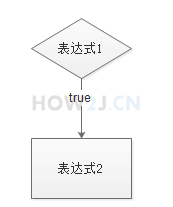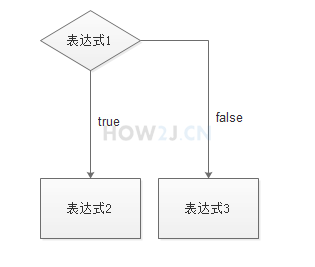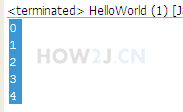# java控制流程教程(九)

2022年4月18日 60点热度 0人点赞 0条评论

# 控制流程系列教材 （一）- Java的If 条件语句

## 示例 1 : if

 `if``(表达式``1``){` `  ``表达式``2``；` `}``public` `class` `HelloWorld {` `    ``public` `static` `void` `main(String[] args) {` `        `  `        ``boolean` `b = ``true``;` `        ``//如果成立就打印yes` `        ``if``(b){` `            ``System.out.println(``"yes"``);` `        ``}` `        `  `    ``}` `}`

## 示例 2 : 多表达式与一个表达式

 `public` `class` `HelloWorld {` `    ``public` `static` `void` `main(String[] args) {` `        `  `        ``boolean` `b = ``false``;` `        ``//如果有多个表达式，必须用大括弧包括起来` `        ``if``(b){` `            ``System.out.println(``"yes1"``);` `            ``System.out.println(``"yes2"``);` `            ``System.out.println(``"yes3"``);` `        ``}` `        `  `        ``//否则表达式2 3 无论b是否为true都会执行` `        `  `        ``if``(b)` `            ``System.out.println(``"yes1"``);` `            ``System.out.println(``"yes2"``);` `            ``System.out.println(``"yes3"``);` `            `  `        ``//如果只有一个表达式可以不用写括弧，看上去会简约一些` `        ``if``(b){` `            ``System.out.println(``"yes1"``);` `        ``}` `        `  `        ``if``(b)` `            ``System.out.println(``"yes1"``);` `        `  `    ``}` `}`

## 示例 3 : if 使用过程中可能遇到的坑

 `public` `class` `HelloWorld {` `    ``public` `static` `void` `main(String[] args) {`   `        ``boolean` `b = ``false``;`   `        ``if` `(b);` `            ``System.out.println(``"yes"``);`   `    ``}` `}`

## 示例 4 : if else

else 代表不成立的情况`public` `class` `HelloWorld {` `    ``public` `static` `void` `main(String[] args) {`   `        ``boolean` `b = ``false``;`   `        ``if` `(b)` `            ``System.out.println(``"yes"``);` `        ``else` `            ``System.out.println(``"no"``);`   `    ``}` `}`

## 示例 5 : else if

else if 是多条件判断

 `public` `class` `HelloWorld {` `    ``public` `static` `void` `main(String[] args) {`   `        ``//如果只使用 if,会执行4次判断` `        ``int` `i = ``2``;` `        ``if` `(i==``1``)` `            ``System.out.println(``1``);` `        ``if` `(i==``2``)` `            ``System.out.println(``2``);` `        ``if` `(i==``3``)` `            ``System.out.println(``3``);` `        ``if` `(i==``4``)` `            ``System.out.println(``4``);` `        `  `        ``//如果使用else if, 一旦在18行，判断成立， 20行和22行的判断就不会执行了，节约了运算资源` `        ``if` `(i==``1``)` `            ``System.out.println(``1``);` `        ``else` `if` `(i==``2``)` `            ``System.out.println(``2``);` `        ``else` `if` `(i==``3``)` `            ``System.out.println(``3``);` `        ``else` `if` `(i==``4``)` `            ``System.out.println(``4``);     ` `        `  `    ``}` `}`

# 控制流程系列教材 （二）- java的switch语句

switch 语句相当于 if else的另一种表达方式

## 示例 1 : switch

switch可以使用byte,short,int,char,String,enum

 `public` `class` `HelloWorld {` `    ``public` `static` `void` `main(String[] args) {` `        `  `        ``//如果使用if else` `        ``int` `day = ``5``;` `        ``if` `(day==``1``)` `            ``System.out.println(``"星期一"``);` `             `  `        ``else` `if` `(day==``2``)` `            ``System.out.println(``"星期二"``);` `        ``else` `if` `(day==``3``)` `            ``System.out.println(``"星期三"``);` `        ``else` `if` `(day==``4``)` `            ``System.out.println(``"星期四"``);` `        ``else` `if` `(day==``5``)` `            ``System.out.println(``"星期五"``);` `        ``else` `if` `(day==``6``)` `            ``System.out.println(``"星期六"``);` `        ``else` `if` `(day==``7``)` `            ``System.out.println(``"星期天"``);` `        ``else` `            ``System.out.println(``"这个是什么鬼？"``);` `        `  `        ``//如果使用switch` `        ``switch``(day){` `            ``case` `1``:` `                ``System.out.println(``"星期一"``);` `                ``break``;` `            ``case` `2``:` `                ``System.out.println(``"星期二"``);` `                ``break``;` `            ``case` `3``:` `                ``System.out.println(``"星期三"``);` `                ``break``;` `            ``case` `4``:` `                ``System.out.println(``"星期四"``);` `                ``break``;` `            ``case` `5``:` `                ``System.out.println(``"星期五"``);` `                ``break``;` `            ``case` `6``:` `                ``System.out.println(``"星期六"``);` `                ``break``;` `            ``case` `7``:` `                ``System.out.println(``"星期天"``);` `                ``break``;` `            ``default``:` `                ``System.out.println(``"这个是什么鬼？"``);` `        ``}` `        `  `    ``}` `}`

# 控制流程系列教材 （三）- java的while语句

while和do-while循环语句

## 示例 1 : 条件为true时 重复执行`public` `class` `HelloWorld {` `    ``public` `static` `void` `main(String[] args) {` `        `  `        ``//打印0到4    ` `        ``int` `i = ``0``;` `        ``while``(i<``5``){` `            ``System.out.println(i);` `            ``i++;` `        ``}` `    ``}` `}`

## 示例 2 : 条件为true时 重复执行，至少会执行一次

 `do``{` `} ``while` `循环``public` `class` `HelloWorld {` `    ``public` `static` `void` `main(String[] args) {` `        `  `        ``//打印0到4` `        ``//与while的区别是，无论是否成立，先执行一次，再进行判断` `        ``int` `i = ``0``;` `        ``do``{` `            ``System.out.println(i);` `            ``i++;           ` `        ``} ``while``(i<``5``);` `        `  `    ``}` `}`zdc1228@163.com